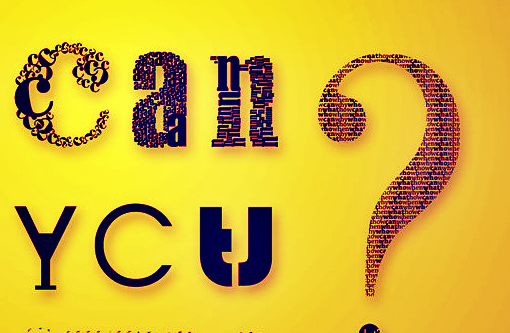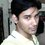# Can you?$$\textit Have\quad you\quad ever\quad exploited\quad symbolic\quad notation\quad of\quad Mathematics?$$

Can you prove ?

$\Huge\sqrt { { x }^{ 2 } } =\left| x \right|\quad \quad \quad ...(1)$

If yes, then read further else skip this note.

Do you know what is 'Scalar Product of two vectors'?

• Scalar product of two vectors: For vectors $\displaystyle \overrightarrow { A }$ and $\displaystyle \overrightarrow { B }$, the scalar product is defined by $\displaystyle \overrightarrow { A } .\overrightarrow { B } = AB\cos { \theta }$ where $\displaystyle { \theta }$ is the angle, in radians with $\displaystyle 0\le \theta \le \pi$ between $\overrightarrow { A }$ and $\displaystyle \overrightarrow { B }$. This is a scalar quantity; that is, a real number, not a vector. The scalar product has the following property:

$\overrightarrow { A } .\overrightarrow { B } =\overrightarrow { B } .\overrightarrow { A } \\ \overrightarrow { A } .\overrightarrow { A } ={ \left| \overrightarrow { A } \right| }^{ 2 }={ A }^{ 2 }\\ \overrightarrow { A } .\left( \overrightarrow { B } +\overrightarrow { C } \right) =\overrightarrow { A } .\overrightarrow { B } +\overrightarrow { A } .\overrightarrow { C }$

Now, the climax:

If $\displaystyle \overrightarrow { A }$ and $\overrightarrow { B }$ are two vectors having an angle $\theta$ between them[ $\theta$ being the smaller angle]

then,

The magnitude of the sum of two vectors is written as

$\displaystyle \left| \overrightarrow { A } +\overrightarrow { B } \right| \quad \quad \quad ...(2)$

using (1), equation (2) can be written as

$\displaystyle \sqrt { { { \left( \overrightarrow { A } +\overrightarrow { B } \right) }^{ 2 } } } =\left| \overrightarrow { A } +\overrightarrow { B } \right|$

or

$\displaystyle \left| \overrightarrow { A } +\overrightarrow { B } \right| = \sqrt { { (\overrightarrow { A } +\overrightarrow { B } ) }{ (\overrightarrow { A } +\overrightarrow { B } ) } } =\sqrt { \overrightarrow { A } .\overrightarrow { A } +2\overrightarrow { A } .\overrightarrow { B } +\overrightarrow { B } .\overrightarrow { B } }$

Therefore,

$\displaystyle \left| \overrightarrow { A } +\overrightarrow { B } \right| =\sqrt { { A }^{ 2 }{ +2AB\cos { \theta } +B }^{ 2 } }$. TaDa! This is the expression for the magnitude of sum of two vectors. Same thing can be derived geometrically.You can find the geometric prove in any standard textbooks.

I found this when I was confused whether 2 is the coefficient of $AB\cos { \theta }$ or not. More significant is the message that symbolic notation in Mathematics is not just to threaten students. Sometimes they help us understand things in simple shorter ways.

Have you ever exploited symbolic notation of Mathematics? Share an example.

[Try to be as elementary as possible]

Ta-Ta.Note by Soumo Mukherjee
6 years, 8 months ago

This discussion board is a place to discuss our Daily Challenges and the math and science related to those challenges. Explanations are more than just a solution — they should explain the steps and thinking strategies that you used to obtain the solution. Comments should further the discussion of math and science.

When posting on Brilliant:

• Use the emojis to react to an explanation, whether you're congratulating a job well done , or just really confused .
• Ask specific questions about the challenge or the steps in somebody's explanation. Well-posed questions can add a lot to the discussion, but posting "I don't understand!" doesn't help anyone.
• Try to contribute something new to the discussion, whether it is an extension, generalization or other idea related to the challenge.

MarkdownAppears as
*italics* or _italics_ italics
**bold** or __bold__ bold
- bulleted- list
• bulleted
• list
1. numbered2. list
1. numbered
2. list
Note: you must add a full line of space before and after lists for them to show up correctly
paragraph 1paragraph 2

paragraph 1

paragraph 2

[example link](https://brilliant.org)example link
> This is a quote
This is a quote
    # I indented these lines
# 4 spaces, and now they show
# up as a code block.

print "hello world"
# I indented these lines
# 4 spaces, and now they show
# up as a code block.

print "hello world"
MathAppears as
Remember to wrap math in $$ ... $$ or $ ... $ to ensure proper formatting.
2 \times 3 $2 \times 3$
2^{34} $2^{34}$
a_{i-1} $a_{i-1}$
\frac{2}{3} $\frac{2}{3}$
\sqrt{2} $\sqrt{2}$
\sum_{i=1}^3 $\sum_{i=1}^3$
\sin \theta $\sin \theta$
\boxed{123} $\boxed{123}$